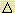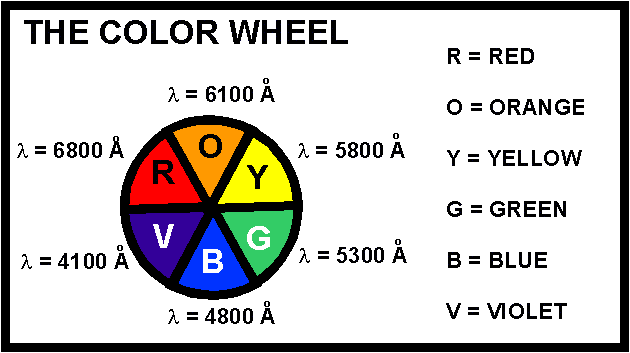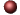Coordination CompoundsExamples ofMultiple Choice Questions

1.
The ______ sphere is enclosed in brackets in formulas for complex species, and it includes the central metal ion plus the coordinated groups.
(a) ligand
(b) donor
(c) oxidation
(d) coordination
(e) chelating

2.
In coordination chemistry, the donor atom of a ligand is
(a) a Lewis acid.
(b) the counter ion
(c) the central metal atom.
(d) the atom in the ligand that shares an electron pair with the metal.
(e) the atom in the ligand that accepts a share in an electron pair from the metal.

3.
Consider the coordination compound, Na2[Pt(CN)4]. The Lewis acid is
(a) [Pt(CN)4]2-
(b) Na+
(c) Pt
(d) Pt2+
(e) CN-

4.
Consider the coordination compound, K2[Cu(CN)4]. A coordinate covalent bond exists between
(a) K+ and CN-
(b) Cu2+ and CN-
(c) K+ and [Cu(CN)4]2-
(d) C and N in CN-
(e) K+ and Cu2+

5.
Given the list of ligands and their corresponding names, choose the pair that disagree.
 LIGAND NAME (a) OH- hydroxo (b) CN- cyanide (c) Cl- chloro (d) H2O aqua (e) NH3 ammine

6.
Select the correct IUPAC name for: [FeF4(OH2)2]-
(a) diaquatetrafluoroiron(III) ion
(b) diaquatetrafluoroferrate(III) ion
(c) diaquatetrafluoroiron(I) ion
(d) diaquatetrafluoroferrate(I) ion
(e) none of these

7.
Select the correct IUPAC name for: [Co(NH3)6]2+
(a) hexammoniacobaltate(II) ion
(b) hexaamminecobaltate(II) ion
(c) hexammoniacobalt(II) ion
(d) hexaamminecobalt(II) ion
(e) hexammoniacobalt ion

8.
Which name-formula combination is NOT correct?
 FORMULA NAME (a) [Co(NH3)4(OH2)I]SO4 tetraammineaquaiodocobalt(III) sulfate (b) K[Cr(NH3)2Cl4] potassium diamminetetrachlorochromate(III) (c) [Mn(CN)5]2- pentacyanomanganate(II) ion (d) [Ni(CO)4] tetracarbonylnickel(0) (e) Ca[PtCl4] calcium tetrachloroplatinate(II)

9.
What is the oxidation number of the central metal atom in the coordination compound
[Pt(NH3)3Cl]Cl?
(a) -1
(b) 0
(c) +1
(d) +2
(e) +3

10.
(Valance Bond Theory) Magnetic measurements indicate that [Co(OH2)6]2+ has 3 unpaired electrons. Therefore, the hybridization of the metal's orbitals in [Co(OH2)6]2+ is:
(a) sp3
(b) sp2d
(c) dsp2
(d) sp3d2
(e) d2sp3

11.
Which one of the following complexes can exhibit geometrical isomerism?
 (a) [Pt(NH3)2Cl2] (square planar) (b) [Zn(NH3)2Cl2] (tetrahedral) (c) [Cu(NH3)4]2+ (square planar) (d) [Co(NH3)5Cl]2+ (octahedral) (e) [Cu(CN)2]- (linear)

12.
A molecule that cannot be superimposed on its mirror image is said to exhibit which of the following?
(a) geometrical isomerism
(b) optical isomerism
(d) reactive isomerism
(e) coordination isomerism

13.
In which one of the following species does the transition metal ion have d3 electronic configuration?
(a) [Cr(NH3)6]3+
(b) [Co(OH2)6]2+
(c) [CoF6]3-
(d) [Fe(CN)6]3-
(e) [Ni(OH2)6]2+

14.
(Valence Bond Theory) The coordination complex, [Cu(OH2)6]2+ has one unpaired electron. Which of the following statements are true?

(1) The complex is octahedral.
(2) The complex is an outer orbital complex.
(3) The complex is d2sp3 hybridized.
(4) The complex is diamagnetic.
(5) The coordination number is 6.

(a) 1, 4
(b) 1, 2, 5
(c) 2, 3, 5
(d) 2, 3
(e) 4, 5

15.
(Crystal Field Theory) Which one of the following statements is FALSE?
(a) In an octahedral crystal field, the d electrons on a metal ion occupy the eg set of orbitals before they occupy the t2g set of orbitals.
(b) Diamagnetic metal ions cannot have an odd number of electrons.
(c) Low spin complexes can be paramagnetic.
(d) In high spin octahedral complexes,oct is less than the electron pairing energy, and is relatively very small.
(e) Low spin complexes contain strong field ligands.

16.
(Crystal Field Theory) When the valence d orbitals of the central metal ion are split in energy in an octahedral ligand field, which orbitals are raised least in energy?
(a) dxy and dx2-y2
(b) dxy, dxz and dyz
(c) dxz and dyz
(d) dxz, dyz and dz2
(e) dx2-y2 and dz2

17.
(Crystal Field Theory) How many unpaired electrons are there in a strong field iron(II) octahedral complex?
(a) 0
(b) 1
(c) 2
(d) 4
(e) 6

18.
(Crystal Field Theory) Consider the complex ion [Mn(OH2)6]2+ with 5 unpaired electrons. Which response includes all the following statements that are true, and no false statements?

I. It is diamagnetic.
II. It is a low spin complex.
III. The metal ion is a d5 ion.
IV. The ligands are weak field ligands.
V. It is octahedral.

(a) I, II
(b) III, IV, V
(c) I, IV
(d) II, V
(e) III, IV

19.
(Crystal Field Theory) Consider the violet-colored compound, [Cr(OH2)6]Cl3 and the yellow compound, [Cr(NH3)6]Cl3. Which of the following statements is false?(a) Both chromium metal ions are paramagnetic with 3 unpaired electrons.
(b)oct for [Cr(NH3)6]3+ is calculated directly from the energy of yellow light.
(c)oct for [Cr(OH2)6]3+ is less thanoct for [Cr(NH3)6]3+.
(d) A solution of [Cr(OH2)6]Cl3 transmits light with an approximate wavelength range of 4000 - 4200 angstroms.
(e) The two complexes absorb their complementary colors.

20.
(Crystal Field Theory) Strong field ligands such as CN-:
(a) usually produce high spin complexes and small crystal field splittings.
(b) usually produce low spin complexes and small crystal field splittings.
(c) usually produce low spin complexes and high crystal field splittings.
(d) usually produce high spin complexes and high crystal field splittings.
(e) cannot form low spin complexes.

1. (d) 2. (d) 3. (d) 4. (b) 5. (b) 6. (b) 7. (d) 8. (c) 9. (d) 10. (d) 11. (a) 12. (b) 13. (a) 14. (b) 15. (a) 16. (b) 17. (a) 18. (b) 19. (b) 20. (c)Click here to return to the top.Choose your next chapter:
Fundamentals of Chemistry | Chemical Formulas & Composition Stoichiometry | Chemical Equations & Rxn Stoichiometry | Types of Chemical Reactions |
| Atomic Structure | Chemical Periodicity | Chemical Bonding | Molecular Structure/Covalent Bonding Theories| Molecular Orbital Theory |
| Acids/Bases/Salts - Theory & Rxns | Acids/Bases/Salts - Calculations (including balancing redox rxns) | Gases | Solids & Liquids | Solutions |
| Thermodynamics | Kinetics | Equilibrium | Aqueous Equilibrium - Acids/Bases/Salts | Aqueous Equilibrium - Buffers & Titrations |
| Aqueous Equilibrium - Slightly Soluble Salts | Electrochemistry | Metallurgy | Metal Properties & Rxns | Nonmetals & Metalloids |
| Coordination Compounds | Nuclear Chemistry | Organic Chem - Formulas/Names/Properties | Organic Chem - Shapes/Rxns/Biopolymers |To report any corrections, please e-mail Dr. Wendy Keeney-Kennicutt.